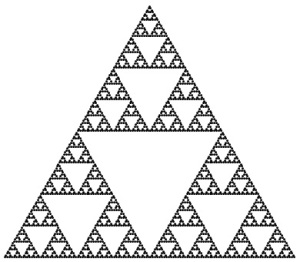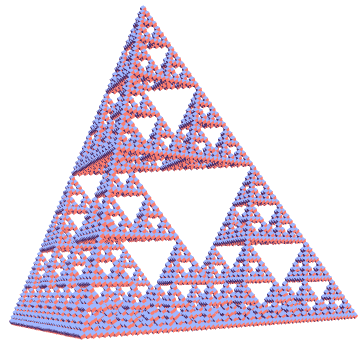# Sierpinski triangle

The Sierpinski triangle is a surprisingly ubiquitous mathematical object. Rather than describing what a Sierpinski triangle is, I may as well show you a picture of one.Essentially, it consists of three identical copies of itself, scaled by a factor of ½. This means that any reasonable definition (e.g. Hausdorff) gives the dimension of the Sierpinski triangle as$\dfrac{\log{3}}{\log{2}}$. We can ask more detailed quantitative questions  about the Sierpinski triangle:

### Moment of inertia

This is quite an easy one, actually. If a Sierpinski triangle of mass m and side length l is rotated about an axis passing normally through its centre, dimensional analysis shows that the moment of inertia is given by$k m l^2$ for some dimensionless quantity k.

Using the recursive definition of the Sierpinski triangle and invoking the Huygens-Steiner theorem (you may know this as the parallel-axis theorem), we get a relation which can then be rearranged to give$k = \frac{1}{9}$. So, the moment of inertia is simply$I = \frac{1}{9} ml^2$.

From this, it is trivial to find the average squared distance between two points:$\frac{2}{9} l^2$, also by Huygens-Steiner.

### Mean distance

Finding the mean (unsquared) distance between two randomly-chosen points is much more non-trivial, and the result is due to Andreas Hanz. It transpires that the mean distance is$\dfrac{466}{885} l$, and the variance of the distance is$904808318/14448151575 l^2$. These results were determined by taking the limit (as the number of discs tends to infinity) of the (normalised) mean distance between two randomly chosen configurations in Tower of Hanoi puzzle.

### Sierpinski tetrahedron

This is an obvious generalisation of the Sierpinski triangle. It is, however, easier to construct: Let x,y,z be real numbers (which are not dyadic rationals) in the interval [0,1]. Then, (x,y,z) is in the Sierpinski tetrahedron if$x \oplus y = z$, where that operator XORs each bit of the binary expansion of the numbers. From this construction, it is easy to deduce that the Hausdorff dimension is precisely 2.This entry was posted in Uncategorized. Bookmark the permalink.

### 8 Responses to Sierpinski triangle

1.rphv says:

Great post! Here’s some code to recursively draw a Sierpinski triangle in JavaScript:
https://rphv.net/computers/sierpinski.html

•apgoucher says:

I had a look at your JavaScript code; it’s elegant and easy to follow. I used Mathematica, naturally, and functional programming as opposed to imperative programming:

Graphics[Point[Nest[Flatten[Map[{#/2 + {0, 2}, #/2 + {Sqrt, -1}, #/2 + {-Sqrt, -1}} &, #], 1] &, {{0, 0}}, 7]]]

2.Hello says:

“Any reasonable definition”

Does this mean that the notion of “topological dimension” is unreasonable?

•apgoucher says:

Consider the set of points in the unit square. If you give it the subspace topology of R^2, it has a Lebesgue covering dimension of 2. If, instead, you give it the topology of the Hilbert curve, it has a Lebesgue covering dimension of 1. So, the topological dimension depends on how you define open sets within the figure.

•Hello says:

Of course the definition depends on the topology. Nevertheless, if you want to talk about the distance between two points later in the article, then you’ve already selected a topology to use.

I’m not saying that the Hausdorff dimension’s not the best, I’m merely arguing that giving it a dimension of 2 isn’t unreasonable.

•apgoucher says:

Yes, I suppose so.

3.時計 ローレックス says:

時計 海外

4.Catisfluffy says:

Does the Sierpinski tetrahedron have well-defined area?